# Team:Leiden/Measurement

<!DOCTYPE html> DOPLLOCK iGEM Leiden

# Measurement

To achieve tight gene regulation in our DOPL LOCK system, we have developed a method to calibrate transcriptional activity of the inducible promoter pBAD with a constitutive promoter p2547. We improved previous calibrations by using the constitutive promoter as an internal reference which minimized the measurement error. Furthermore, we quantified the activities of the promoters by fitting the data to a logistic equation. Our results allow future iGEM teams to predict and regulate their genetic circuits when using pBAD and Anderson’s promoter family. Our calibration method of promoters can easily be applied to all iGEM projects that require precise gene regulation and improving the standardization of the promoters in the Registry.

# Overview

One of the major goals of our project is to balance the amount of toxin and antitoxin in bacterial cells. In the ideal scenario, certain amounts of toxin would be inhibited by corresponding amounts of antitoxin. When the antitoxin amount is reduced because of degradation of the inducer or horizontal gene transfer, the toxin would be rapidly released and cell growth would be inhibited. Ideally, all toxin and antitoxin genes should be regulated by different constitutive promoters to extend the balance phase. However, the combination of constitutive promoters is hard to define as literature has been found insufficient to provide an appropriate toxin:antitoxin ratio.

In order to clarify this balance, we designed our system to use inducible promoters at first to find the balance phase of the toxin-antitoxin (TA) system rapidly (see the Experiments page for more information). Then, we would replace the inducible promoters with specific constitutive promoters to reduce the complexity of the system. Therefore, comparisons of expression levels between constitutive promoter and inducible promoter were required.

Even though the transcriptional activities of promoters we used (BBa_I0500, pBAD and constitutive promoter family of BBa_J23100) have been characterized with fluorescent proteins previously, only the calibration of Anderson's promoter family was done by fluoresceins (iGEM2019_Hamburg). Therefore, their transcriptional activities are incomparable because of the different measurement units (arbitrary unit, AU) used in different research. Moreover, the regulating capability of the same promoter also differs in different cellular environments. To solve this, we designed experiments to calibrate inducible promoter pBAD with constitutive promoter BBa_J23100 (referred to as p2547 in the following). A similar calibration method has been proposed by Sanches-Medeiros et al., where they calibrated the transcriptional activity of the lac promoter with multiple constitutive promoters .

In our experiments, we have made constructs of pBAD::mCherry and p2547::mCherry in the pJUMP49 plasmid (the full construct can be seen in the Parts page). The plasmids were transformed into the TOP10 E. coli strain. Subsequently, L-arabinose was added when optical density at 600 nm (OD600) was equal to 0.5 followed by a fluorescence measurement overnight. The constant mCherry expression of the p2547 was used as the calibration unit of pBAD. Using p2547 as the internal reference, the deviation of fluorescence caused by different strain, different growth conditions and different measuring equipment can be minimized. Our measurement time included the lag phase, exponential phase and the stationary phase of the bacteria growth. Therefore, the fluorescence curve can be fitted to a logistic function and the maximum fluorescence can be used to quantify the transcriptional activity.

Our measurements are highly reproducible, as the use of a reference constitutive promoter standardizes the variation of gene expression caused by different strains, growth conditions and other gene components. The result of the pBAD calibration provides future iGEM teams with information on choosing promoters to achieve the desired regulation of synthetic gene-circuits. Our calibration method for correlating inducible promoters with constitutive promoters can apply to all constitutive and inducible promoters within the iGEM registry.

• # Equipment

1. Spectrophotometer
2. 1 and 2mL Micro-centrifuge tubes
3. 20 ml Falcon tube
4. Multichannel pipet
5. Shaking incubator
6. Plate reader (TECAN spark, with fluorescence cassette)
7. Flat bottomed 96 wells plate with lid
• # Reagents

1. LB medium
2. L-Arabinose stock solution (dissolved in LB; 20%, w/v)
3. Antibiotics stock solution (50mg/ml)

# Equipment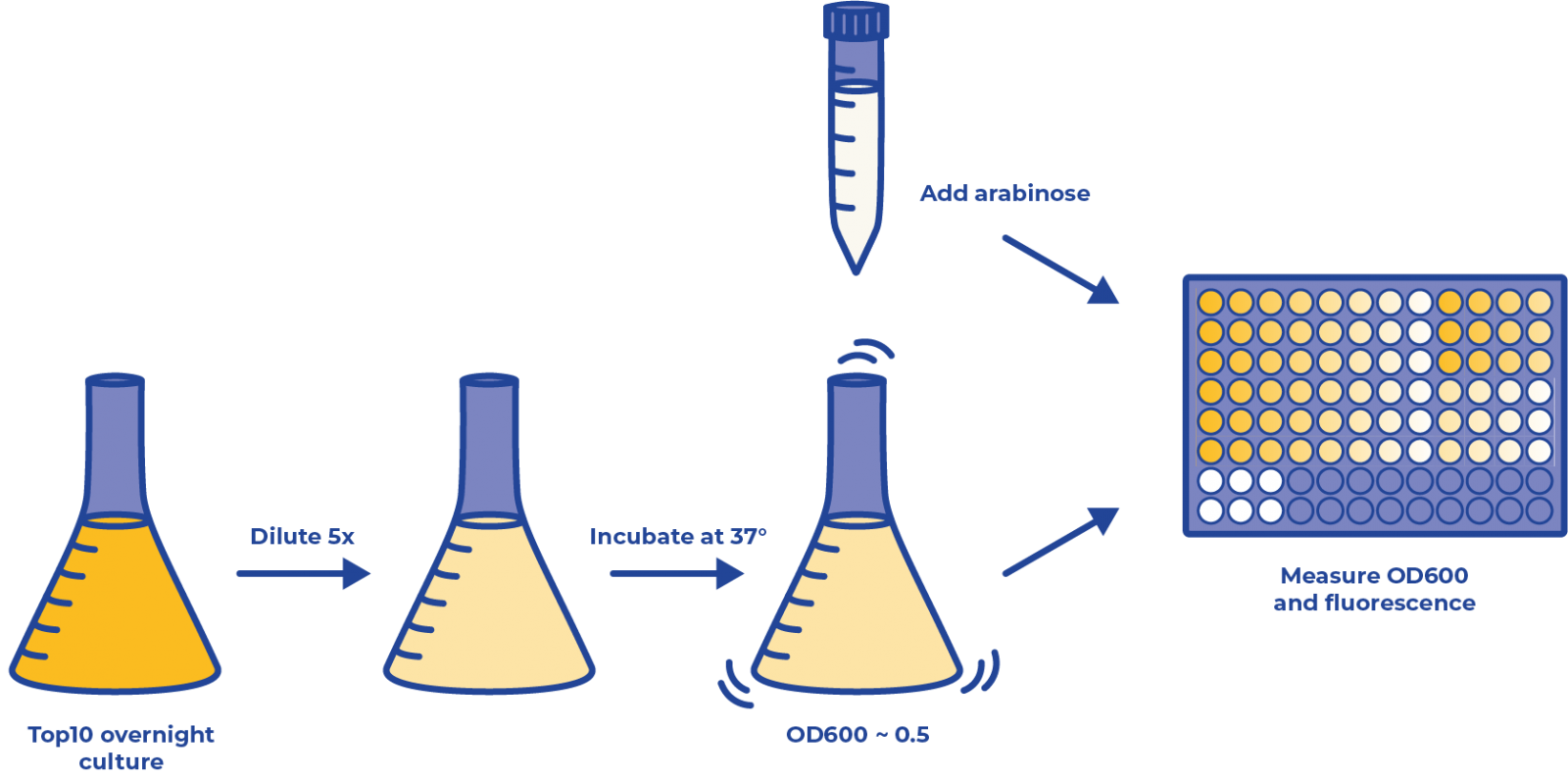Figure 1: The overview of experiment procedures for promoter calibration.

1. Prepare two experiment strains (one transformed with plasmid containing the construct of inducible promoter::fluorescent protein, the other with constitutive promoter::fluorescent protein) and a control strain (transformed with plasmid only containing inducible promoter but no further construct) in LB medium and incubate them at 37°C overnight.
2. Dilute the overnight culture 5 times.
3. Incubate the culture in the shaker for approximately 1 h until their OD600 reaches 0.5.

💡 Tips: Bacteria are in exponential growth phase at OD=0.5. In this state, their metabolism is fully functioning. The pathways that are not critical to their survival, such as synthetic protein expression, are also active.

1. Add 190μL of the diluted bacteria liquid culture to each well in a 96-well plate and 10μL of inducer stock solution (concentrations are shown below). The strain transformed with only the inducible promoter was used as a negative control. This strain was treated with the same arabinose concentration as pBAD::mCherry strain. LB mediums with ampicillin (final concentration = 100 mg/ml) were used as blank control.

💡 Tips: We suggest minimizing the time slot between adding inducer and measuring. In our case we calculated and prepared a series of stock solution (20%, 4%, 0.8%, 0.16%, 0.032%, 0.0064%, 0.000128%) in advance to transfer and make final concentration (1%, 0.2%, 0.04%, 0.008%, 0.0016%, 0.00032%, 0.000064%) in 96-well plate.

The plate layout in our project is shown below: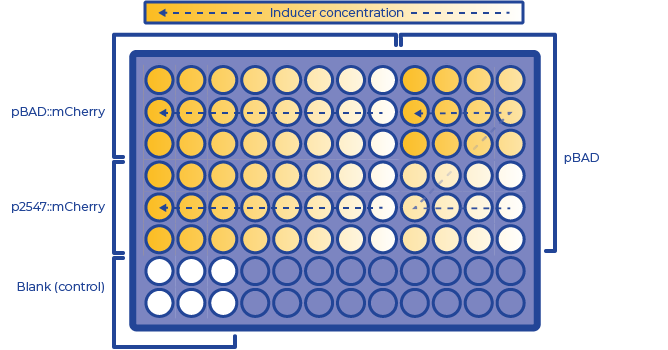Figure 2: The layout of 96-well plate for promoter calibration

Control Setting: The p2547::mCherry strain could be regarded as the positive control of pBAD::mCherry strain. We also used this pBAD strain as a negative control as they helped to exclude the influence of pBAD cassette to fluorescence. LB medium with corresponding antibiotics was used as a blank control.

1. Put the 96-well plate in the plate reader for the measurement of fluorescence. The temperature was maintained at 37 °C and the plate was shaken at 120 rpm. The measurement was done every 12 min.

## Data analysis

The data is analyzed using R studio. Detailed procedure and code can be found in: Plate reader data analysis.txt

In summary, we first normalized fluorescence intensity to OD. Then the data is fitted to a standard form of the logistic equation common in ecology and evolution :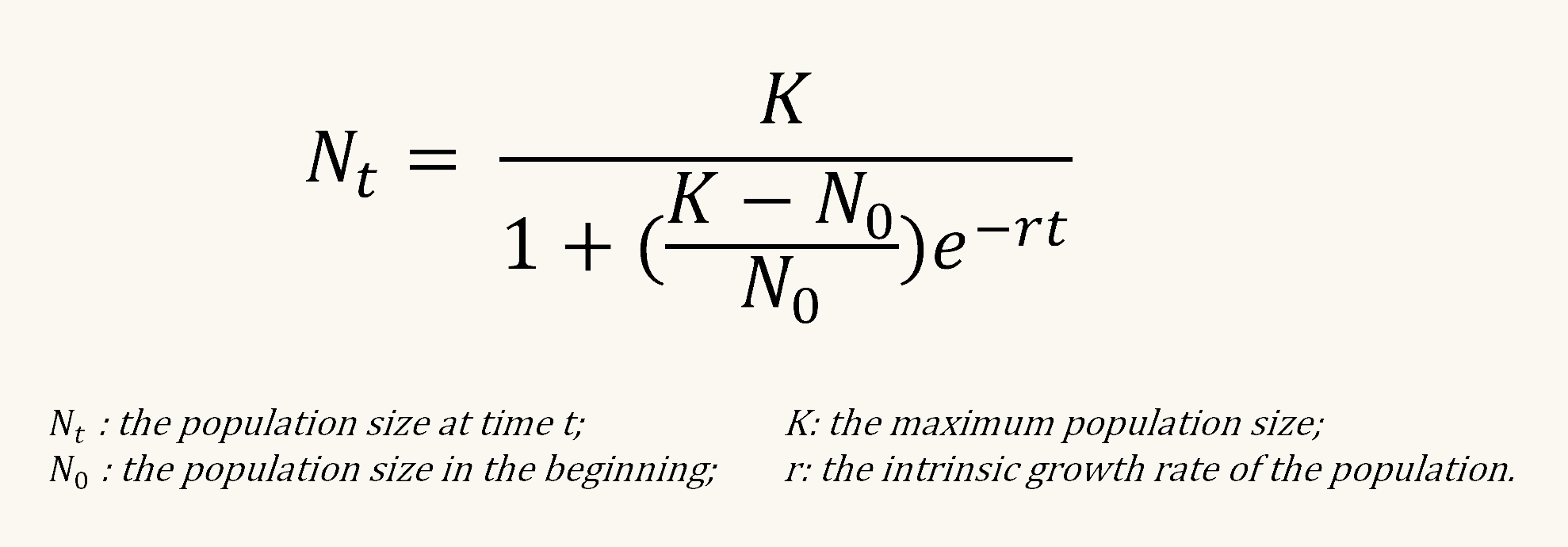Then, we calculated the ratio of maximum fluorescence produced by an inducible promoter (pBAD in our case) compared to a constitutive promoter (p2547 in our case). The relative fluorescence and the logarithm of arabinose concentration was finally fitted into linear regression.

The data is analyzed using R studio. Detailed procedure and code can be found in below:

• # Analyzing with R

``````library(ggplot2)
library(reshape2)
library(ggplot2)
library(growthcurver)
library(purrr)
library(ggpubr)
library(tidyr)``````
``library(readxl)``

# Making tidy data

``````# Read data
# Make data tidy
OD600 <- gather(Plate_reader_OD600, "position", "OD600", 3:98)
# Combine all table
table1 <- cbind(mCherry, OD600[,4])
Row <- substr(table1\$position, 1, 1)
Column <- as.numeric(substr(table1\$position, 2, 3))
table2 <- cbind(table1, Row, Column)``````

``````ABC <- c(1, 0.2, 0.04, 0.008, 0.0016, 0.00032, 0.000064, 0, 1, 0.2, 0.04, 0.008)
arabinose <- rep(ABC, times = 3, each = 98)
CDE <- c(1,0.2,0.04,0.008,0.0016,0.00032,0.000064,0,0.0016,0.00032,0.000064,0)
arabinose <- c(arabinose, rep(CDE, times = 3, each = 98))
GH <- c(1,0.2,0.04,0.008,0.0016,0.00032,0.000064,0,0,0,0,0)
arabinose <- c(arabinose, rep(GH, times = 2, each = 98))
table3 <- cbind(table2, arabinose)``````

# Function for return standard deviation

``````data_summary <- function(data, varname, groupnames){
require(plyr)
summary_func <- function(x, col){
c(mean = mean(x[[col]], na.rm=TRUE),
sd = sd(x[[col]], na.rm=TRUE))
}
data_sum<-ddply(data, groupnames, .fun=summary_func,
varname)
data_sum <- rename(data_sum, c("mean" = varname))
return(data_sum)
}``````

# Divide experiment group

``library(dplyr)``
``````PBad <- filter(table3, Row=="A"|Row=="B"|Row=="C", Column < 9)
P2547 <- filter(table3, Row=="D"|Row=="E"|Row=="F", Column < 9)
P1 <- filter(table3, Row!="G"&Row!="H", Column > 8)
NC <- filter(table3, Row == "G" | Row == "H", Column < 9)
BC <- filter(table3, Row == "G" | Row == "H", Column > 8)``````

# Caculate sd

``library(plyr)``
``````PBad_mCherry <- data_summary(PBad, varname="mCherry", groupnames = c("arabinose","Time"))
P2547_mCherry <- data_summary(P2547, varname="mCherry", groupnames = c("arabinose","Time"))
P1_mCherry <- data_summary(P1, varname="mCherry", groupnames = c("arabinose","Time"))
NC_mCherry <- data_summary(NC, varname="mCherry", groupnames = c("arabinose","Time"))
BC_mCherry <- data_summary(BC, varname="mCherry", groupnames = c("arabinose","Time"))``````

# Check OD600

``````PBad\$arabinose <- as.factor(PBad\$arabinose)
p1 <- ggplot(filter(PBad,Column<5), aes(x=Time, y=OD600, group = position, color = arabinose)) +
geom_line() +
geom_point() +
theme_bw() +
theme(axis.text.x = element_text(angle=90, hjust=0))
p1``````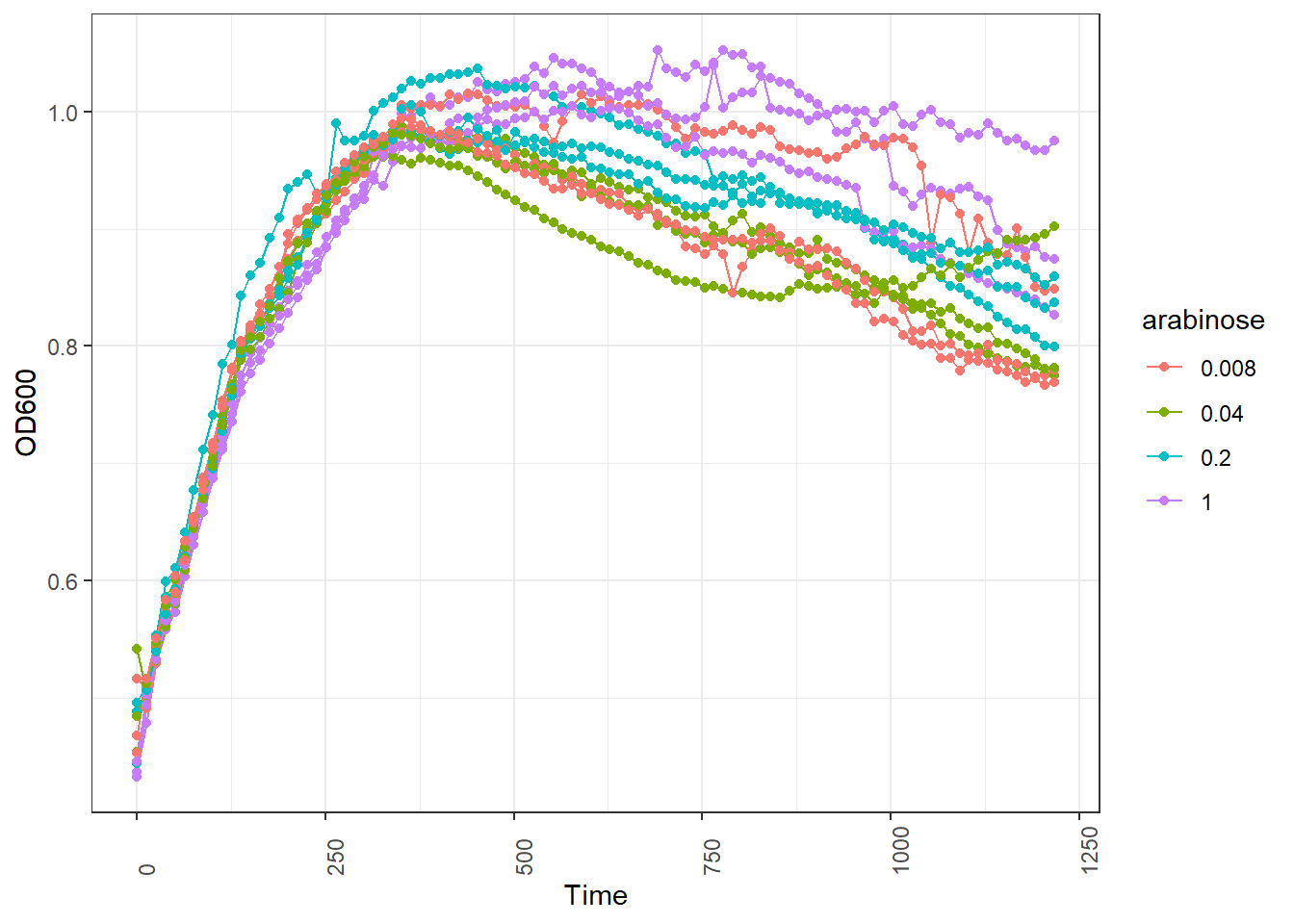``````p2 <- ggplot(filter(PBad,Column>4), aes(x=Time, y=OD600, group = position, color = arabinose)) +
geom_line() +
geom_point() +
theme_bw() +
theme(axis.text.x = element_text(angle=90, hjust=0))
p2``````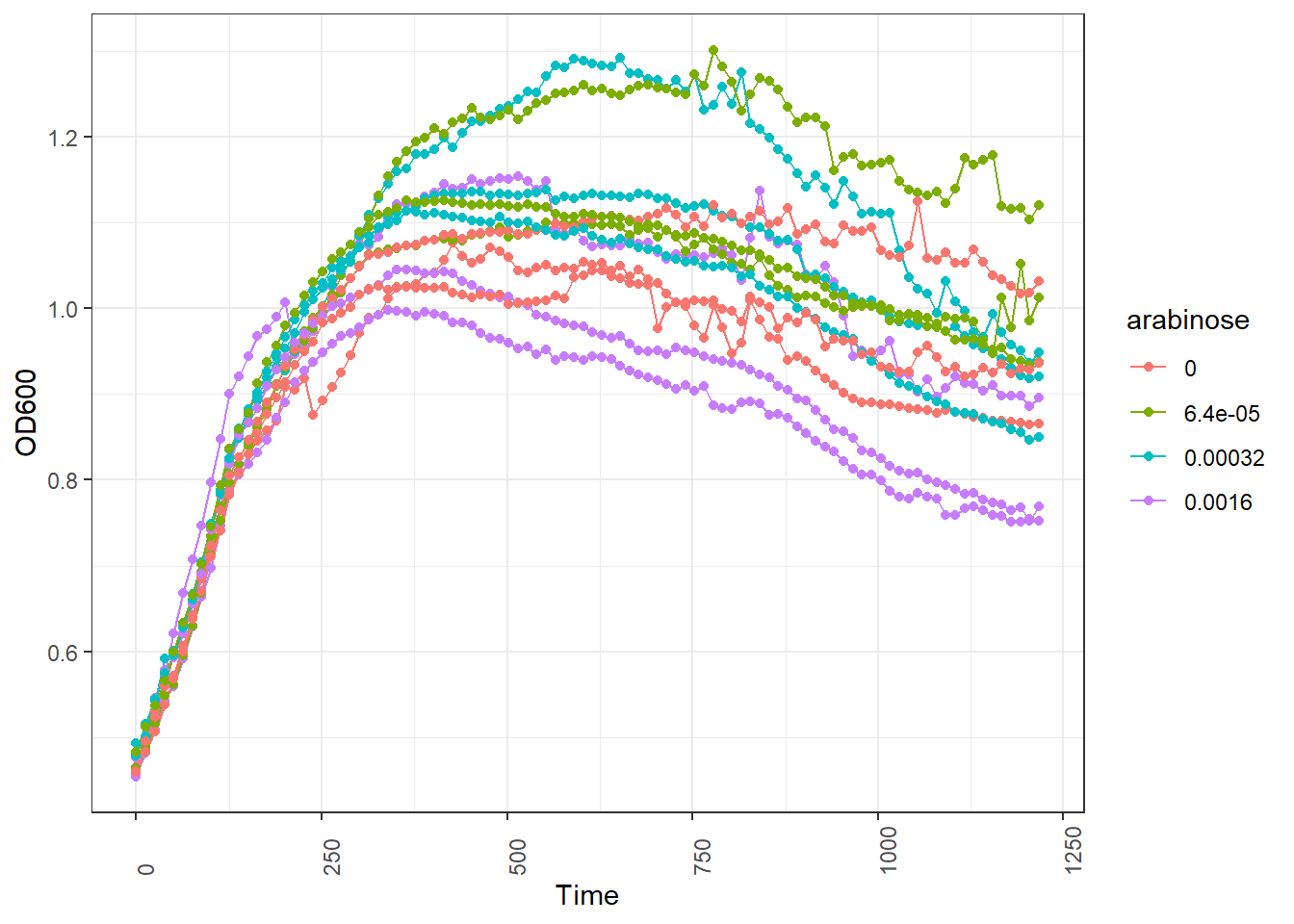# Check data quality

## Blank control

LB medium with only antibiotics

``````BC_plot <- ggplot(BC_mCherry, aes(x=Time, y=mCherry, group = arabinose, color = arabinose)) +
geom_line() +
geom_point() +
theme_bw() +
theme(axis.text.x = element_text(angle=90, hjust=0)) +
geom_errorbar(aes(ymin=mCherry-sd, ymax=mCherry+sd), width=.2)
BC_plot``````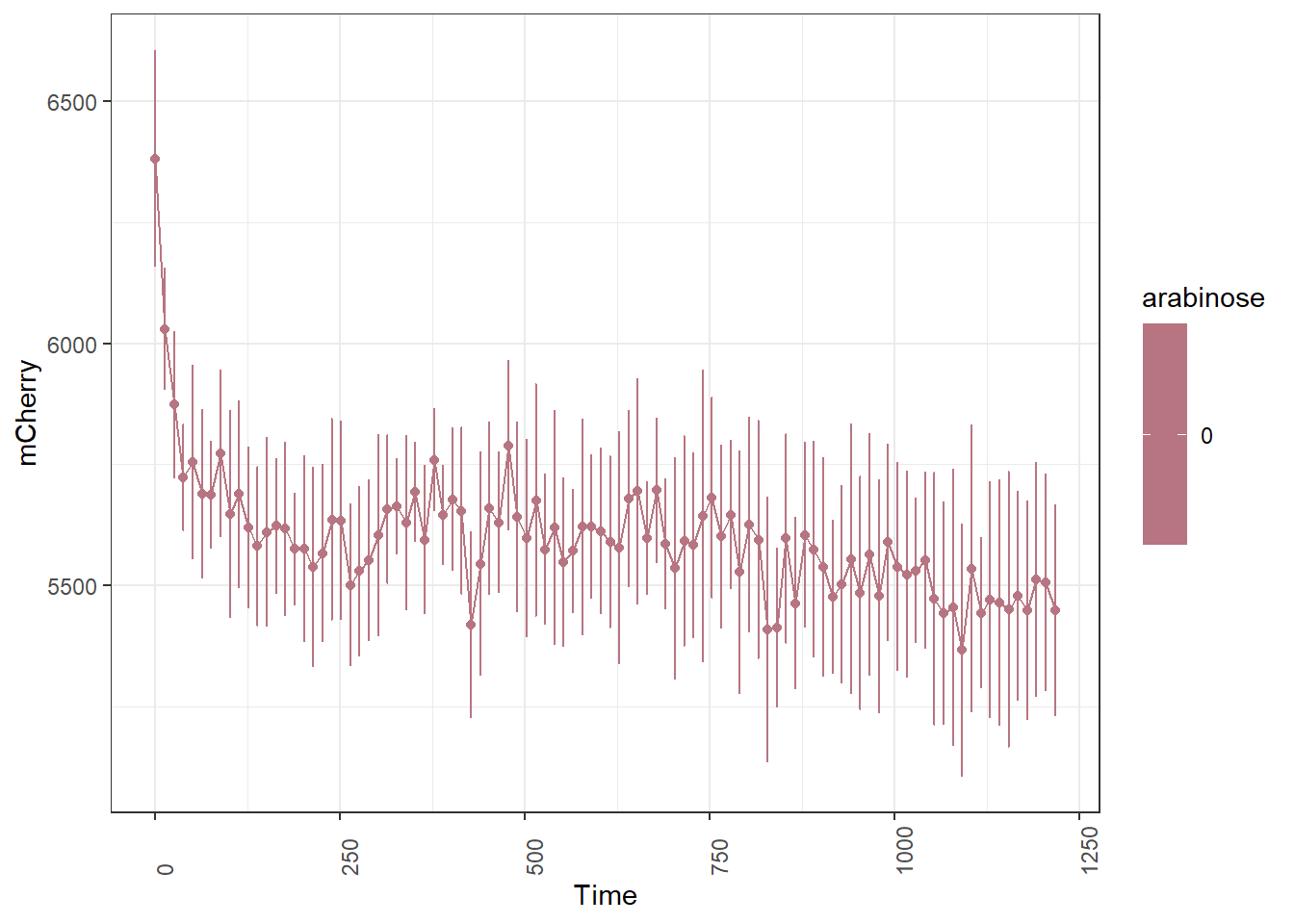## Negative control

TOP strain in LB medium. Same arabinose gradiant with triplicate

``````NC_plot <- ggplot(NC_mCherry, aes(x=Time, y=mCherry, group = arabinose, color = arabinose)) +
geom_line() +
geom_point() +
theme_bw() +
theme(axis.text.x = element_text(angle=90, hjust=0)) +
geom_errorbar(aes(ymin=mCherry-sd, ymax=mCherry+sd), width=.2)
NC_plot``````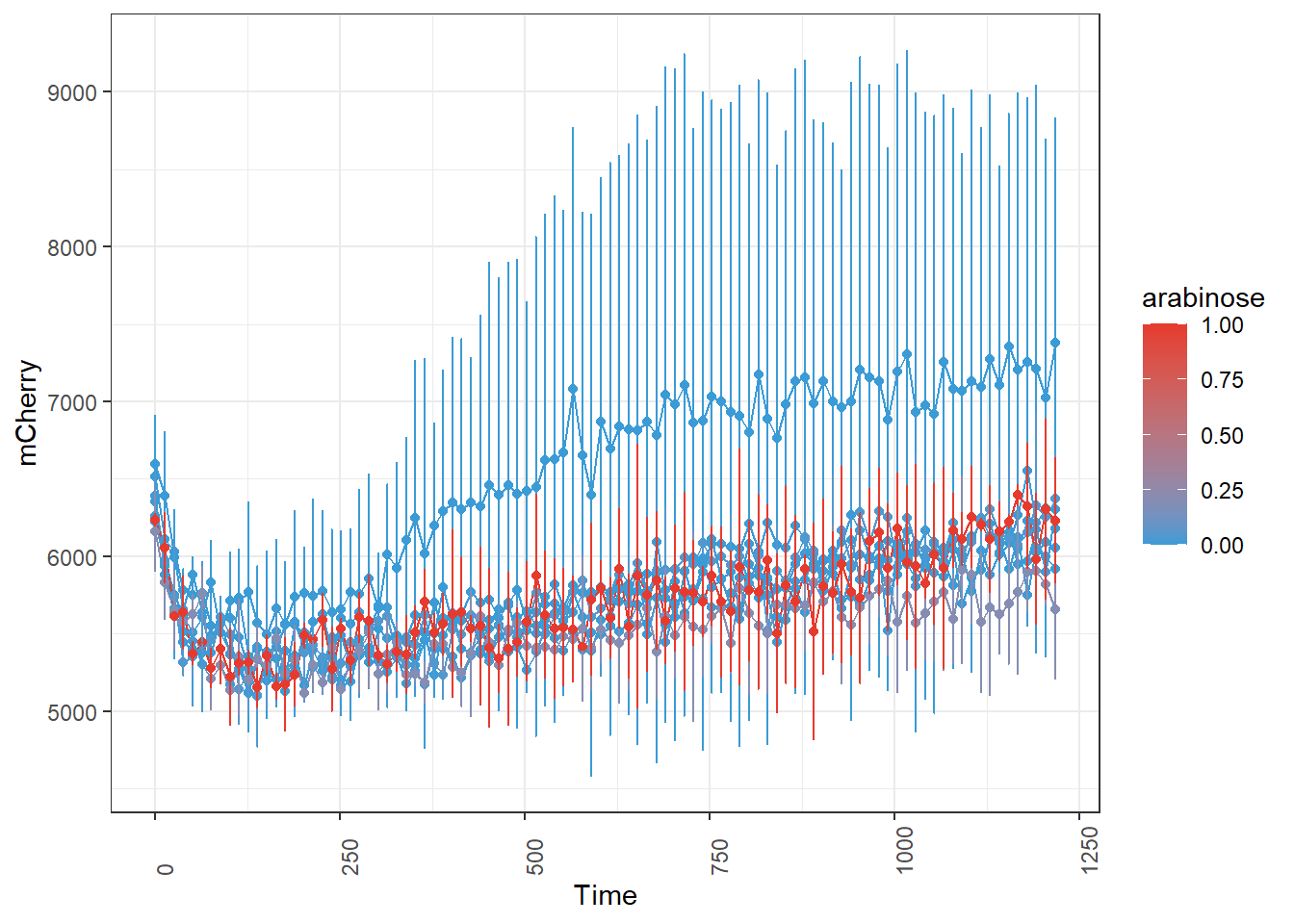## P1-mCherry

TOP10 strain with plasmid P1-mCherry cassette.

``````c <- ggplot(P1_mCherry, aes(x=Time, y=mCherry, group = arabinose, color = arabinose)) +
geom_line() +
geom_point() +
theme_bw() +
theme(axis.text.x = element_text(angle=90, hjust=0)) +
geom_errorbar(aes(ymin=mCherry-sd, ymax=mCherry+sd), width=.2)
c``````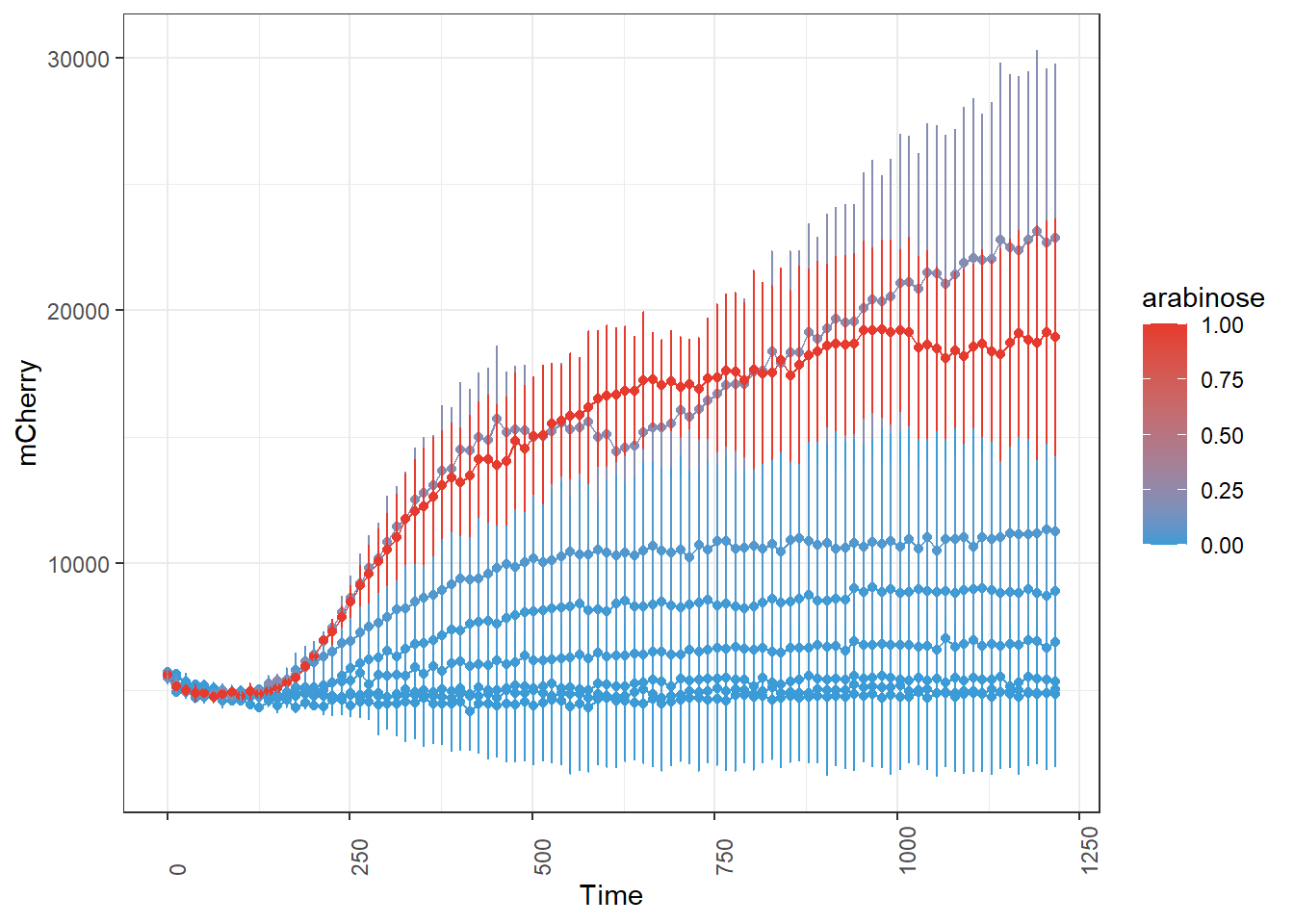## P2547-mCherry

TOP10 strain with plasmid P2547-mCherry cassette.

``````P2547_plot <- ggplot(P2547_mCherry, aes(x=Time, y=mCherry, group = arabinose, color = arabinose)) +
geom_line() +
geom_point() +
theme_bw() +
theme(axis.text.x = element_text(angle=90, hjust=0)) +